2．序列极限存在的判别法

[柯西准则] 序列{xn}的极限存在的充分必要条件是：对任意给定的ε>0，都存在正整数N=N(ε)，使得当n>N时，不等式

|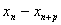|<ε

[上下极限相等] 序列{xn}的极限（有穷或无穷）存在的充分必要条件是：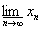=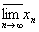[单调有界] 单调有界序列必有极限.

[有有界变差]* 有有界变差序列(即存在正数c，使得|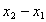|+||+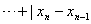|<c,n=2,3,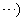必有极限.

[序列对比] 若序列{xn}满足条件ynxnzn，且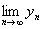=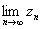=c，则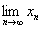=c

[施笃兹定理] 对序列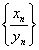,(i)nn0(n0为某一自然数)时，yn+1>yn(ii)=+,(iii)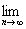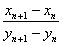=l (有限数或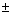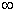)，则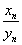==l

[加权平均序列] wnk0(k =1,2,n;n=1,2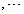),=1,对固定的k,wnk=0.如果xn=a,=a

* 对于函数有有界变差是这样定义的：假定f (x)[a,b]上有限，在[a,b]上作分点a=x0<x1<…

<xn-1<xn=b,作和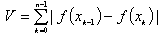,V的上确界叫做f (x)[a,b]上的全变差，记为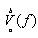.